maths > vector-algebra

Cross Product: First Principles

what you'll learn...

Overview

Vector Cross Product : First Principles

»  multiplied with component in perpendicular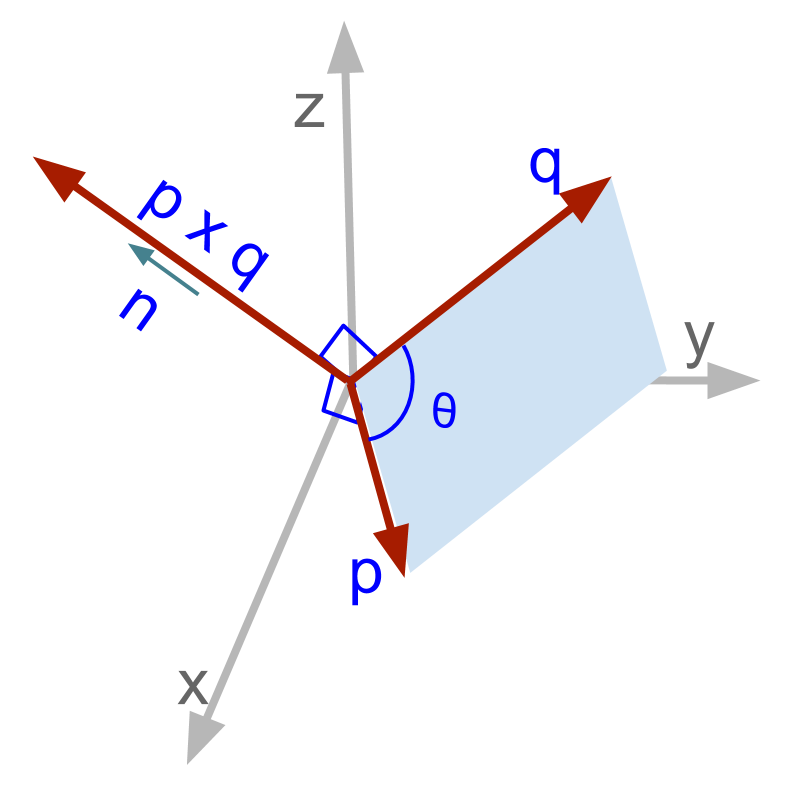→  $\stackrel{\to }{p}×\stackrel{\to }{q}$$\vec{p} \times \vec{q}$ $=\stackrel{\to }{p}×\stackrel{\to }{b}$$= \vec{p} \times \vec{b}$ $=|\stackrel{\to }{p}||\stackrel{\to }{b}|\stackrel{^}{n}$$= | \vec{p} | | \vec{b} | \hat{n}$

→  product of magnitudes of components in perpendicular

→  direction perpendicular to both the vectors

in perpendicular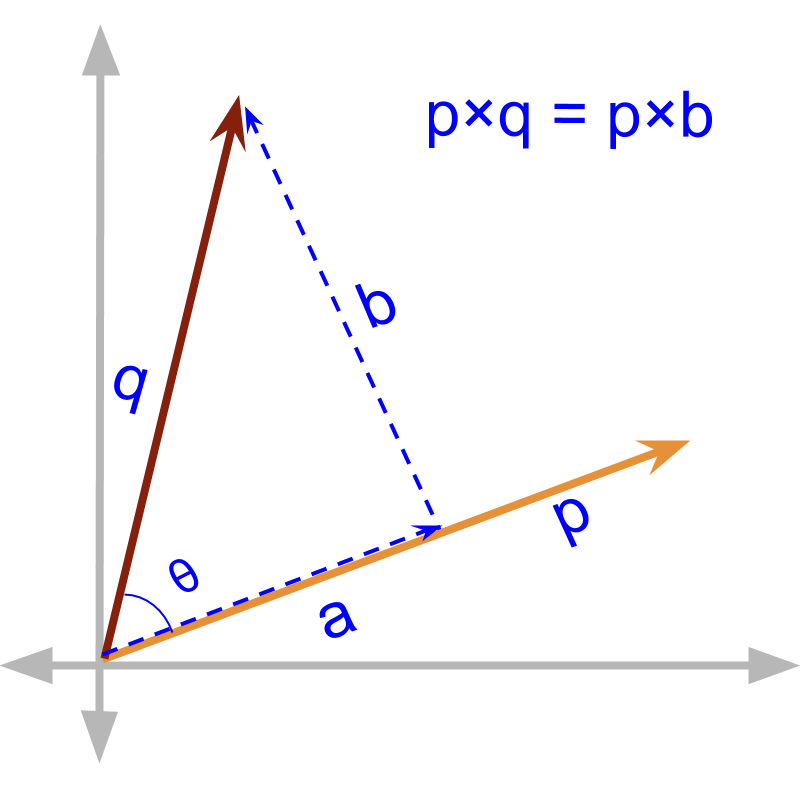The product where vector components in perpendicular interact is defined. Given vectors $\stackrel{\to }{p}$$\vec{p}$ and $\stackrel{\to }{q}$$\vec{q}$ as shown in figure. The component of $\stackrel{\to }{q}$$\vec{q}$ perpendicular to the $\stackrel{\to }{p}$$\vec{p}$ us $\stackrel{\to }{b}$$\vec{b}$

The angle between the vectors is $\theta$$\theta$. Then $|\stackrel{\to }{b}|$$| \vec{b} |$ $=|q|\mathrm{sin}\theta$$= | q | \sin \theta$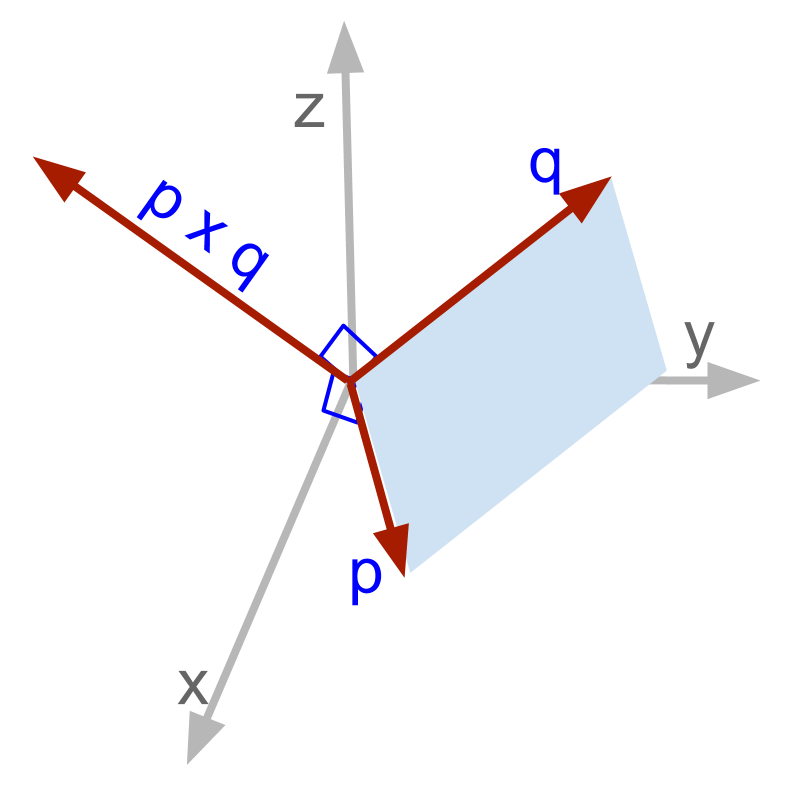What will be a good choice of direction of the product between components in perpendicular?

Two intersecting lines, which are not parallel, define a plane. The normal on the face of the plane describes the plane and so, the normal is taken as the direction of the product.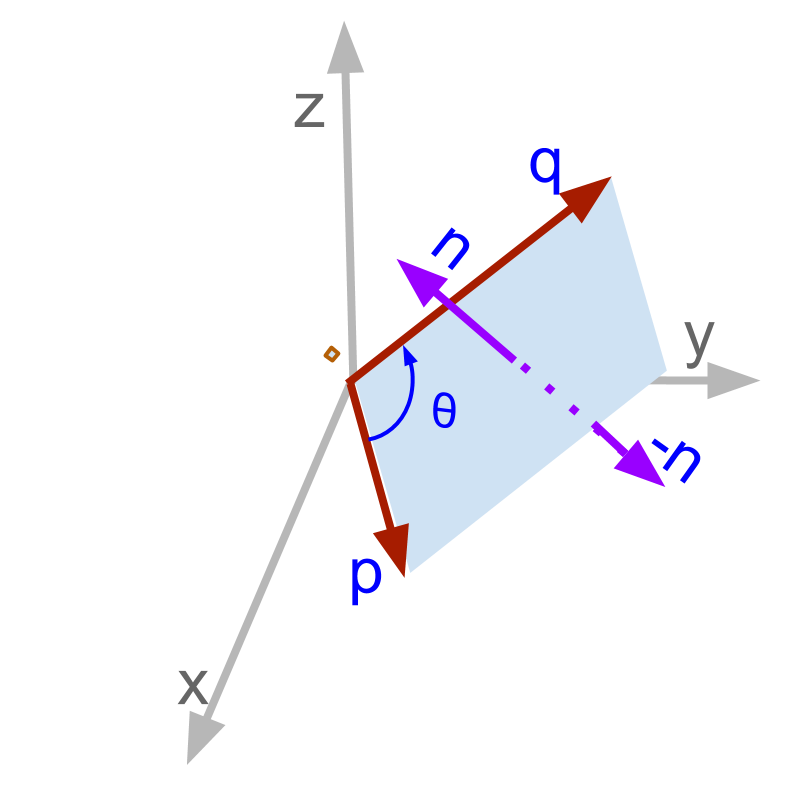A plane can be described by

•  normal which is one side of the plane, or

•  the negative of the normal, which is the other side of the plane.

One of this can be chosen as the direction of cross product $\stackrel{\to }{p}×\stackrel{\to }{q}$$\vec{p} \times \vec{q}$.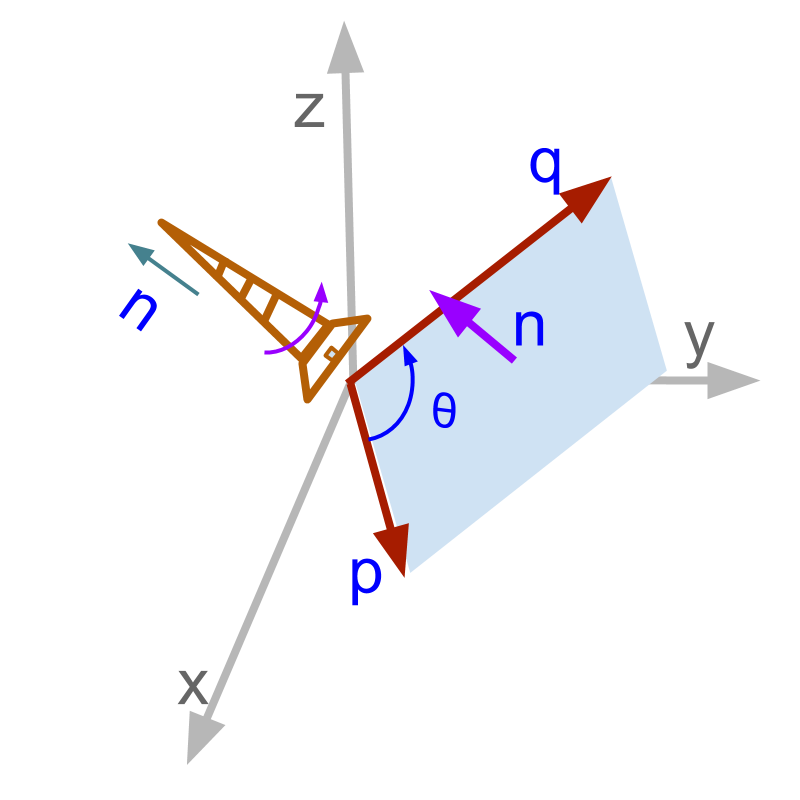The direction of the cross product $\stackrel{\to }{p}×\stackrel{\to }{q}$$\vec{p} \times \vec{q}$ is shown in the figure. In a standard right-handed rectangular coordinate system,
The direction in which a standard screw advances when it turns from $\stackrel{\to }{p}$$\vec{p}$ to $\stackrel{\to }{q}$$\vec{q}$, defines the normal. s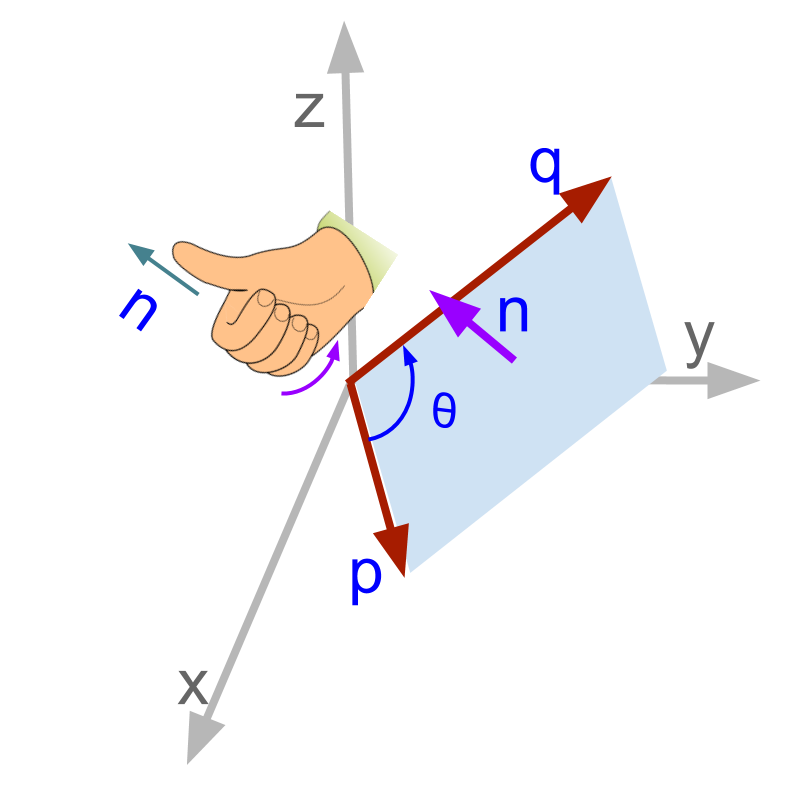Another form to find the direction of the cross product $\stackrel{\to }{p}×\stackrel{\to }{q}$$\vec{p} \times \vec{q}$ is shown in the figure. In a standard right-handed rectangular coordinate system,
The direction pointed by the right thumb when fingers are curled to point from $\stackrel{\to }{p}$$\vec{p}$ to $\stackrel{\to }{q}$$\vec{q}$, defines the normal.Formal definition of vector cross product.
$\stackrel{\to }{p}×\stackrel{\to }{q}=|p||q|\mathrm{sin}\theta \stackrel{^}{n}$$\vec{p} \times \vec{q} = | p | | q | \sin \theta \hat{n}$ where $\stackrel{^}{n}$$\hat{n}$ is the normal denoting the direction of right handed rotation from $\stackrel{\to }{p}$$\vec{p}$ to $\stackrel{\to }{q}$$\vec{q}$.

summary

Vector Cross Product: for vectors $\stackrel{\to }{p},\stackrel{\to }{q}\in {R}^{3}$$\vec{p} , \vec{q} \in {\mathbb{R}}^{3}$
$\stackrel{\to }{p}×\stackrel{\to }{q}=\left(|p||q|\mathrm{sin}\theta \right)\stackrel{^}{n}$$\vec{p} \times \vec{q} = \left(| p | | q | \sin \theta\right) \hat{n}$
where $\stackrel{^}{n}$$\hat{n}$ is the unit vector of right-handed rotation from $\stackrel{\to }{p}$$\vec{p}$ to $\stackrel{\to }{q}$$\vec{q}$.

Vector Cross Product is defined as the product of components in perpendicular.

Outline